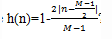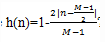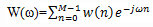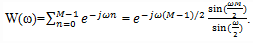Courses

Test: FIR Filters Windows Design - 1

10 Questions MCQ Test Digital Signal Processing | Test: FIR Filters Windows Design - 1

Description
Attempt Test: FIR Filters Windows Design - 1 | 10 questions in 10 minutes | Mock test for Electrical Engineering (EE) preparation | Free important questions MCQ to study Digital Signal Processing for Electrical Engineering (EE) Exam | Download free PDF with solutions
QUESTION: 1

. Which of the following defines the rectangular window function of length M-1?

Solution:

Explanation: We know that the rectangular window of length M-1 is defined as
w(n)= 1, n=0,1,2…M-1
=0, else where.

QUESTION: 2

The multiplication of the window function w(n) with h(n) is equivalent to the multiplication of H(w) and W(w).

Solution:

Explanation: According to the basic formula of convolution, the multiplication of two signals w(n) and h(n) in time domain is equivalent to the convolution of their respective Fourier transforms W(w) and H(w).

QUESTION: 3

What is the width of the main lobe of the frequency response of a rectangular window of length M-1?

Solution:

Explanation: The width of the main lobe width is measured to the first zero of W(ω)) is 4π/M.

QUESTION: 4

The width of each side lobes decreases with an increase in M.

Solution:

Explanation: Since the width of the main lobe is inversely proportional to the value of M, if the value of M increases then the main lobe becomes narrower. In fact, the width of each side lobes decreases with an increase in M.

QUESTION: 5

With an increase in the value of M, the height of each side lobe:

Solution:

Explanation: The height of each side lobes increase with an increase in M such a manner that the area under each side lobe remains invariant to changes in M.

QUESTION: 6

As M is increased, W(ω) becomes wider and the smoothening produced by the W(ω) is increased.

Solution:

Explanation: As M is increased, W(ω) becomes narrower and the smoothening produced by the W(ω) is reduced.

QUESTION: 7

Which of the following windows has a time domain sequenceSolution:

Explanation: The Bartlett window which is also called as triangular window has a time domain sequence as, 0≤n≤M-1.

QUESTION: 8

The width of each side lobes decreases with an decrease in M.

Solution:

Explanation: Since the width of the main lobe is inversely proportional to the value of M, if the value of M increases then the main lobe becomes narrower. In fact, the width of each side lobes decreases with an increase in M.

QUESTION: 9

What is the approximate transition width of main lobe of a Hamming window?

Solution:

Explanation: The transition width of the main lobe in the case of Hamming window is equal to 8π/M where M is the length of the window.

QUESTION: 10

What is the Fourier transform of the rectangular window of length M-1?

Solution:

Explanation: We know that the Fourier transform of a function w(n) is defined asFor a rectangular window, w(n)=1 for n=0,1,2….M-1
Thus we getUse Code STAYHOME200 and get INR 200 additional OFF Use Coupon Code

Track your progress, build streaks, highlight & save important lessons and more!

Similar ContentRelated tests